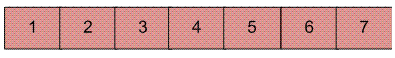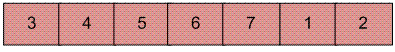# C++ Program for Reversal algorithm for array rotation

• Difficulty Level : Basic
• Last Updated : 28 May, 2022

Write a function rotate(arr[], d, n) that rotates arr[] of size n by d elements.
Example :

```Input :  arr[] = [1, 2, 3, 4, 5, 6, 7]
d = 2
Output : arr[] = [3, 4, 5, 6, 7, 1, 2] ```Rotation of the above array by 2 will make arrayThe first 3 methods to rotate an array by d elements has been discussed in this post.
Method 4 (The Reversal Algorithm) :
Algorithm :

```rotate(arr[], d, n)
reverse(arr[], 1, d) ;
reverse(arr[], d + 1, n);
reverse(arr[], 1, n);```

Let AB are the two parts of the input array where A = arr[0..d-1] and B = arr[d..n-1]. The idea of the algorithm is :

• Reverse A to get ArB, where Ar is reverse of A.
• Reverse B to get ArBr, where Br is reverse of B.
• Reverse all to get (ArBr) r = BA.

Example :
Let the array be arr[] = [1, 2, 3, 4, 5, 6, 7], d =2 and n = 7
A = [1, 2] and B = [3, 4, 5, 6, 7]

• Reverse A, we get ArB = [2, 1, 3, 4, 5, 6, 7]
• Reverse B, we get ArBr = [2, 1, 7, 6, 5, 4, 3]
• Reverse all, we get (ArBr)r = [3, 4, 5, 6, 7, 1, 2]

Below is the implementation of the above approach :

## C++

 `// C++ program for reversal algorithm` `// of array rotation` `#include ` `using` `namespace` `std;`   `/*Function to reverse arr[] from index start to end*/` `void` `reverseArray(``int` `arr[], ``int` `start, ``int` `end)` `{` `    ``while` `(start < end) {` `        ``int` `temp = arr[start];` `        ``arr[start] = arr[end];` `        ``arr[end] = temp;` `        ``start++;` `        ``end--;` `    ``}` `}`   `/* Function to left rotate arr[] of size n by d */` `void` `leftRotate(``int` `arr[], ``int` `d, ``int` `n)` `{` `    ``if` `(d == 0)` `        ``return``;` `    ``// in case the rotating factor is` `    ``// greater than array length` `    ``d = d % n;`   `    ``reverseArray(arr, 0, d - 1);` `    ``reverseArray(arr, d, n - 1);` `    ``reverseArray(arr, 0, n - 1);` `}`   `// Function to print an array` `void` `printArray(``int` `arr[], ``int` `size)` `{` `    ``for` `(``int` `i = 0; i < size; i++)` `        ``cout << arr[i] << ``" "``;` `}`   `/* Driver program to test above functions */` `int` `main()` `{` `    ``int` `arr[] = { 1, 2, 3, 4, 5, 6, 7 };` `    ``int` `n = ``sizeof``(arr) / ``sizeof``(arr);` `    ``int` `d = 2;`   `    ``// Function calling` `    ``leftRotate(arr, d, n);` `    ``printArray(arr, n);`   `    ``return` `0;` `}`

Output :

`3 4 5 6 7 1 2`

Time Complexity: O(N), where N represents the size of the given array.
Auxiliary Space: O(1), no extra space is required, so it is a constant.

Please refer complete article on Reversal algorithm for array rotation for more details!

My Personal Notes arrow_drop_up
Recommended Articles
Page :